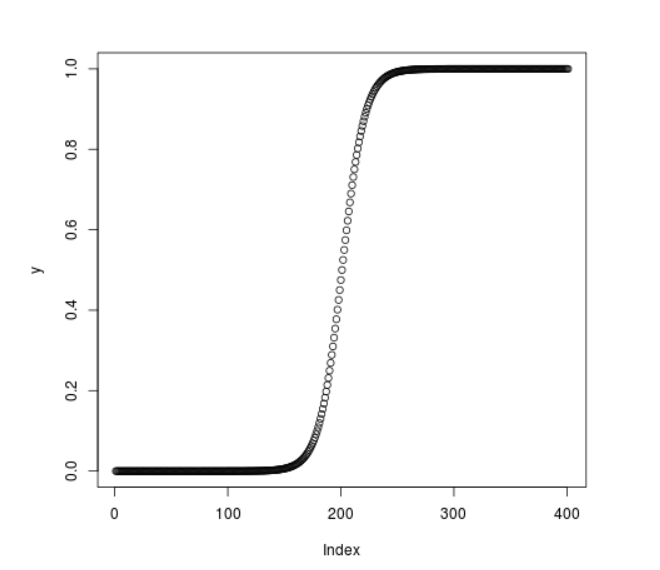# Compute Cumulative Logistic Density in R Programming – plogis() Function

`plogis()` function in R Language is used to compute logistic cumulative density of the distribution. It also creates a plot of the density of the logistic cumulative distribution.

Syntax: plogis(vec)

Parameters:
vec: Vector of x-values for density

Example 1:

 `# R program to calculate  ` `# cumulative logistic density ` ` `  `# Create a vector of x-values ` `x <``-` `seq(``-``1``, ``1``, by ``=` `0.1``) ` ` `  `# Calling the plogis() function ` `y <``-` `plogis(x) ` `y `

Output:

```  0.2689414 0.2890505 0.3100255 0.3318122 0.3543437 0.3775407 0.4013123
 0.4255575 0.4501660 0.4750208 0.5000000 0.5249792 0.5498340 0.5744425
 0.5986877 0.6224593 0.6456563 0.6681878 0.6899745 0.7109495 0.7310586
```

Example 2:

 `# R program to calculate  ` `# cumulative logistic density ` ` `  `# Create a vector of x-values ` `x <``-` `seq(``-``20``, ``20``, by ``=` `0.1``) ` ` `  `# Calling the plogis() function ` `y <``-` `plogis(x) ` ` `  `# Plot the graph ` `plot(y) `

Output:My Personal Notes arrow_drop_upCheck out this Author's contributed articles.

If you like GeeksforGeeks and would like to contribute, you can also write an article using contribute.geeksforgeeks.org or mail your article to contribute@geeksforgeeks.org. See your article appearing on the GeeksforGeeks main page and help other Geeks.

Please Improve this article if you find anything incorrect by clicking on the "Improve Article" button below.

Article Tags :

Be the First to upvote.

Please write to us at contribute@geeksforgeeks.org to report any issue with the above content.